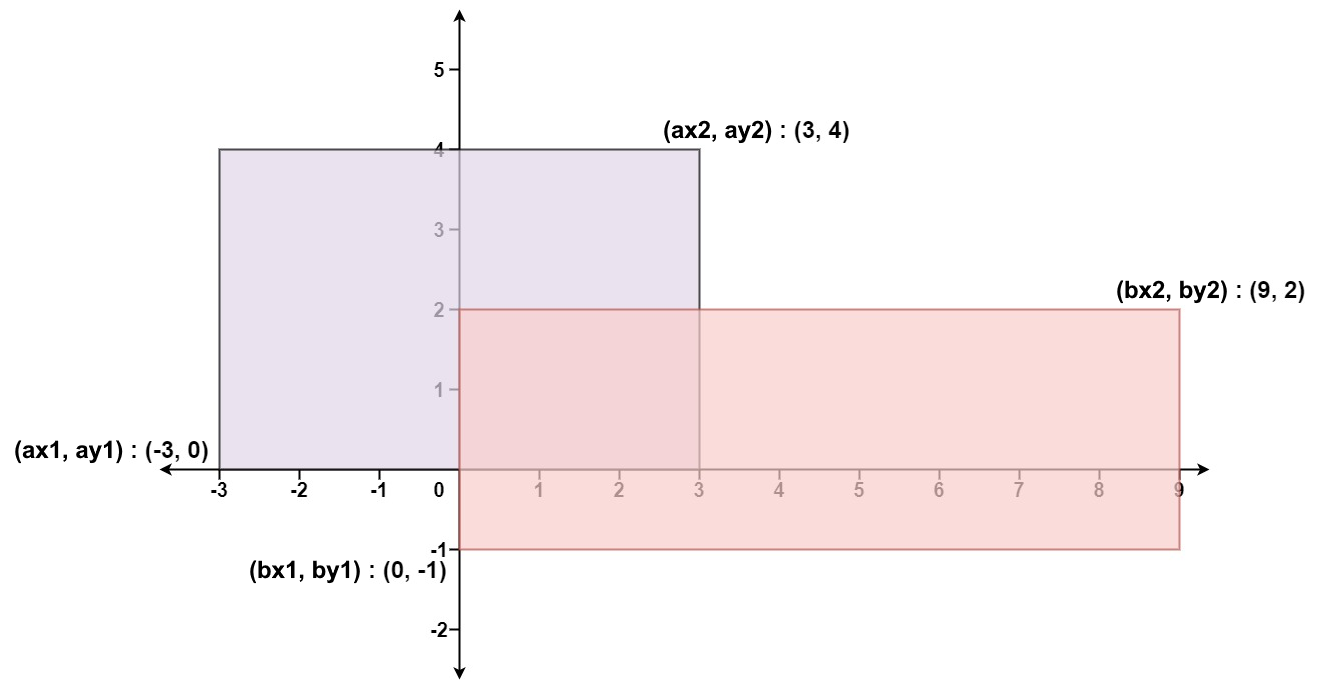# GeetCode Hub

Given the coordinates of two rectilinear rectangles in a 2D plane, return the total area covered by the two rectangles.

The first rectangle is defined by its bottom-left corner `(ax1, ay1)` and its top-right corner `(ax2, ay2)`.

The second rectangle is defined by its bottom-left corner `(bx1, by1)` and its top-right corner `(bx2, by2)`.

Example 1:```Input: ax1 = -3, ay1 = 0, ax2 = 3, ay2 = 4, bx1 = 0, by1 = -1, bx2 = 9, by2 = 2
Output: 45
```

Example 2:

```Input: ax1 = -2, ay1 = -2, ax2 = 2, ay2 = 2, bx1 = -2, by1 = -2, bx2 = 2, by2 = 2
Output: 16
```

Constraints:

• `-104 <= ax1, ay1, ax2, ay2, bx1, by1, bx2, by2 <= 104`

class Solution { public int computeArea(int ax1, int ay1, int ax2, int ay2, int bx1, int by1, int bx2, int by2) { } }